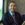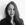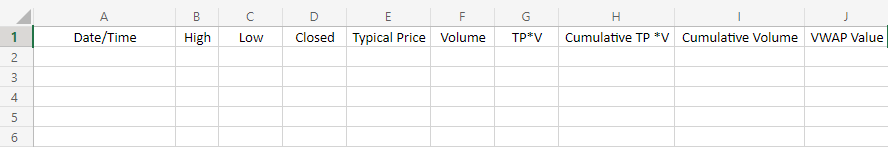# How to Calculate VWAP in Excel

The volume-weighted average price (VWAP) is one of the best measurements you can utilize for your day trading endeavors. So, what's the formula and calculation?By Andrew MoranEdited by Kelli Harris

Published November 30, 2021.

You are a day or swing trader. You want to know more than just moving averages and the Relative Strength Index (RSI). You have come across the volume-weighted average price (VWAP). You want to know the various applications of VWAP.

Well, you come to the right place, whether you want to know the VWAP calculation or the VWAP formula on Excel. Let's explore.

### VWAP Calculation Formula

Here is a step-by-step breakdown of how to calculate the volume-weighted average price:

1. Select your time frame (one minute, five minutes, 15 minutes, etc.)
2. Calculate the typical price by adding the high, low, and close, and dividing by three.
3. Multiple the typical by the volume for that time frame, known as the TPV.
4. Keep track of the running total TPV values and cumulative volume.
5. Calculate the VWAP.

Put simply, you add up the dollars traded for each transaction, multiply the price by the number of shares traded, and then divide the total shares traded.

Here is a VWAP calculation example:

• Price: (H+L+C)/3
• VWAP: (Volume * Price) / Volume

Here is a step-by-step breakdown of how to calculate VWAP on a Microsoft Excel spreadsheet:

• A: Date / Time
• B: High
• C: Low
• D: Closed
• E: Typical Price (TP)
• F: Volume (V)
• G: TP *V
• H: Cumulative TP * V
• I: Cumulative Volume
• J: VWAP Value

Here is a screenshot of what your spreadsheet should look like using a five-minute time frame:﻿

### The VWAP Is Your New Best Friend

Whether you are a novice or seasoned investor, you will realize that the volume-weighted average price technical indicator is your new best friend. This is one of the best complements you can make for your overall investment strategy. Be it a VWAP chart or an Excel spreadsheet, you can exploit the benefits of VWAP to maximize your profits.

﻿